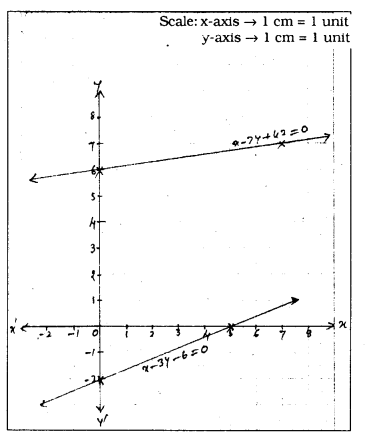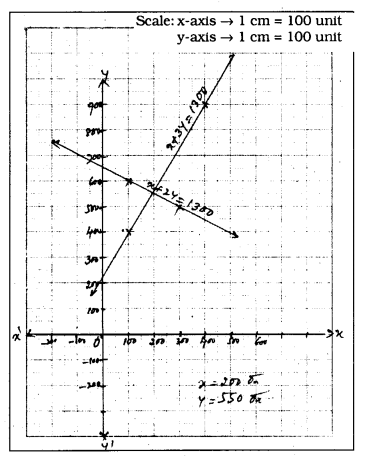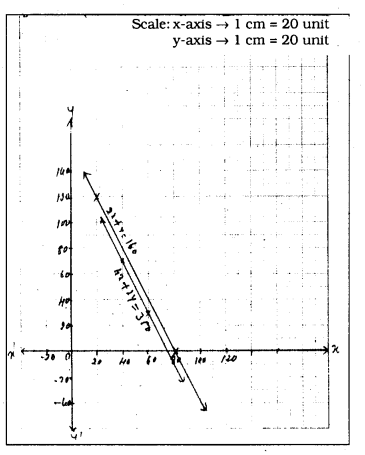# KSEEB SSLC Class 10 Maths Solutions Chapter 3 Pair of Linear Equations in Two Variables Ex 3.1

KSEEB SSLC Class 10 Maths Solutions Chapter 3 Pair of Linear Equations in Two Variables Ex 3.1 are part of KSEEB SSLC Class 10 Maths Solutions. Here we have given Karnataka SSLC Class 10 Maths Solutions Chapter 3 Pair of Linear Equations in Two Variables Exercise 3.1.

## Karnataka SSLC Class 10 Maths Solutions Chapter 3 Pair of Linear Equations in Two Variables Exercise 3.1

Question 1.
Aftab tells his daughter, “Seven years ago, I was, seven times as old as you were then. Also, three years from now, I shall be three times as old as you will be.” (Isn’t this interesting?) Represent this situation algebraically and graphically.
Solution:
Let the present age of father be x’
Let the age of daughter be ‘y’.
Before seven years, age of father is x – 7
Before seven years, age of daughter is y – 7.
Father was 7 times as old as daughter.
∴ x – 7 = 7 (y – 7)
x – 7 = 7y – 49
x – 7y = -49 + 7
x – 7y = – 42
∴ x – 7y + 42 = 0 …………….. (i)
After three years, age of father is x + 3
After three years, age of daughter is y + 3
Three years from now will three times as old as father.
∴ x + 3 = 3(y + 3)
x + 3 = 3y + 9
x – 3y + 3 – 9 = 0
3 – 3y – 6 = 0 ………….. (ii)
∴ Algebraically linear equations :
x – 7y + 42 = 0
x – 3y – 6 =0
If we represent this linear equations through graph, we have
i) x – 7y + 42 = 0
-7y = -x – 42
7y = x + 42
$$\quad y=\frac{x+42}{7}$$

 x 0 7 $$y=\frac{x+42}{7}$$ 6 7

ii) x – 3y – 6 = 0
-3y = -x + 6
3y = x – 6
$$\quad y=\frac{x-6}{3}$$

 x 0 +6 $$y=\frac{x-6}{3}$$ -2 0Question 2.
The coach of a cricket team buys 3 bats and 6 balls for Rs. 3900. Later, she buys another bat and 3 more balls of the same kind for Rs. 1300. Represent this situation algebriacally and geometrically.
Solution:
Let the cost of each bat be Rs. ‘x’
Let the cost of each ball be Rs. ‘y’.
3x + 6y = 3900
∴ x + 2y = 1300 ………. (i)
Cost of another bat and ball be x + 3y = 1300 ………….. (ii)
∴Algebriacally
x + 2y = 1300
x + 3y = 1300
And to represent through graph :
i) x + 2y = 1300
2y = 1300 – x
$$\quad x=\frac{1300-x}{2}$$

 x 100 200 $$y=\frac{1300-x}{2}$$ 600 500

ii) x + 3y = 1300
3y = 1300 – x
$$\quad y=\frac{1300-x}{3}$$

 x 100 400 $$y=\frac{1300-x}{3}$$ 400 900Question 3.
The cost of 2 kg of apples and 1 kg of grapes on a day was found to be Rs. 160. After a month, the cost of 4 kg of apples and 2 kg of grapes is Rs. 300. Represent the situation algebraically and geometrically.
Solution:
Algebraically,
If Cost of each kg. of apple be Rs. ‘x’,
Cost of grapes be Rs. ‘y’. Then
2x + y = 160
4x + 2y = 300
To represent geometrically,
i) 2x + y = 160
y = -2x + 160

 x 20 80 y = 2x + 160 120 0

ii) 4x + 2y = 300
2x + y= 150
y = 150 – 2x

 x 40 60 y = 150 – 2x 70 30We hope the given KSEEB SSLC Class 10 Maths Solutions Chapter 3 Pair of Linear Equations in Two Variables Ex 3.1 will help you. If you have any query regarding Karnataka SSLC Class 10 Maths Solutions Chapter 3 Pair of Linear Equations in Two Variables Exercise 3.1, drop a comment below and we will get back to you at the earliest.